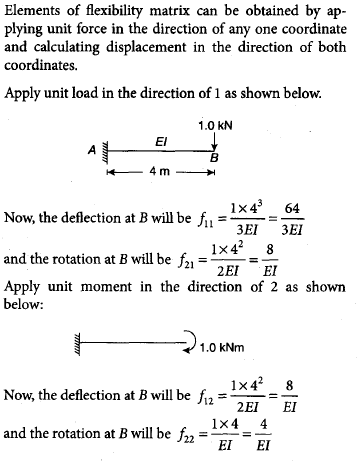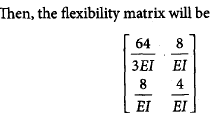Courses

# Structural Analysis - 1

## 15 Questions MCQ Test GATE Civil Engineering (CE) 2022 Mock Test Series | Structural Analysis - 1

Description
This mock test of Structural Analysis - 1 for GATE helps you for every GATE entrance exam. This contains 15 Multiple Choice Questions for GATE Structural Analysis - 1 (mcq) to study with solutions a complete question bank. The solved questions answers in this Structural Analysis - 1 quiz give you a good mix of easy questions and tough questions. GATE students definitely take this Structural Analysis - 1 exercise for a better result in the exam. You can find other Structural Analysis - 1 extra questions, long questions & short questions for GATE on EduRev as well by searching above.
QUESTION: 1

### A beam carries a uniformly distributed load throughout its length. In which of the following configurations will the strain energy be maximum?

Solution:

Strain Energy in beams is basically equals to integration of square of Bending Moment over the length divided by EI.
For a given beam, higher the static indeterminacy lower the bending moment is developed in the cross-section, thus, resulting in lower strain energy.
Moreover, if the indeterminacy is same then, lower the number of supports, higher will be bending moment generated thus, leading to higher strain energy.
From above statements, we can conclude that cantilever has maximum strain energy.

QUESTION: 2

### The force in member AB of the truss shown below is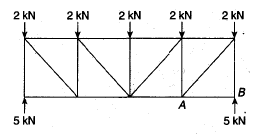Solution:

If we consider the joint equilibrium at B, the force in member AB will be zero.

QUESTION: 3

### The force in member BD of the truss shown below is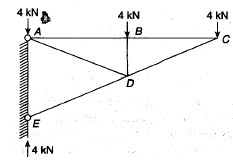Solution:

If we consider the joint equilibrium at b, the force in member BD will be 4 kN compressive.

QUESTION: 4

Which one of the following structures is statically determinate and stable?

Solution: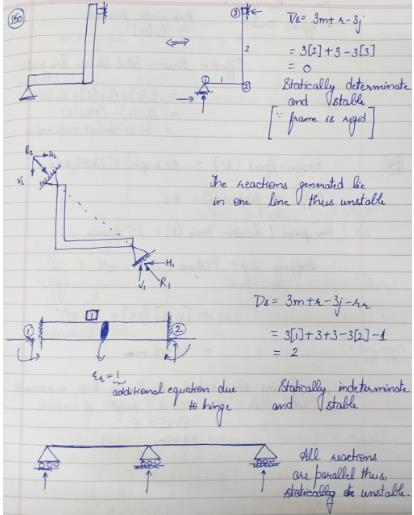QUESTION: 5

For a two-hinged arch, if one of the supports settles down vertically, then the horizontal thrust

Solution:
QUESTION: 6

The number of independent equations to be satisfied for static equilibrium in a space structure is

Solution:

Summation of forces and moments in all three directions need to be zero
Fx=0; Fy=0; Fz=0; Mx=0; My=0; Mz=0

QUESTION: 7

A propped cantilever is acted upon by a moment M0 at the propped end. What is the prop action?

Solution:

A structure is statically determinate when the external reactions can be determined from force-equilibrium equations.
A structure is stable when the whole or part of the structure is prevented from large displacements on account of loading.
In (a), the structure is stable and there are three reaction components that can be determined from two force equilibrium conditions and one moment equilibrium condition.
But in case of (b), the structure is stable but statically indeterminate to the second degree and in case of (c), the structure has both reaction components coinciding with each other, so the moment equilibrium conditions will never be satisfied and the structure will not be under equilibrium.
In (d), the structure is simply unstable.

QUESTION: 8

The ratio of the maximum deflection between a simply supported beam carrying a point load at its midspan and the same beam carrying the seem load as total load of uniformly distributed load will be

Solution: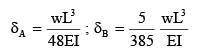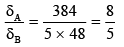QUESTION: 9

For the beam shown in the below figure, to have the maximum positive bending moment equal to negative bending moment, the value of L1 has to be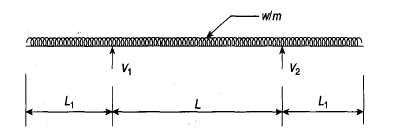Solution: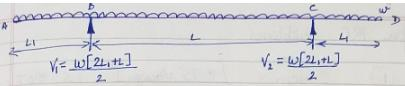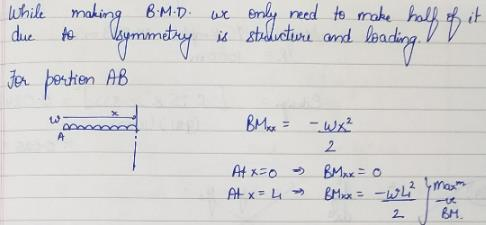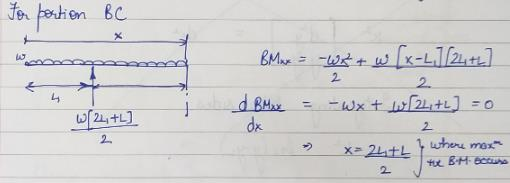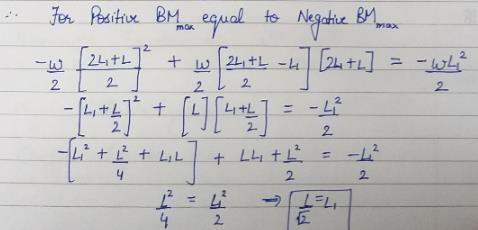QUESTION: 10

A propped cantilever of uniform flexural rigidity is loaded as shown in the given figure. The bending moment at fixed end is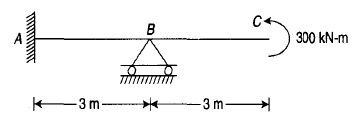Solution: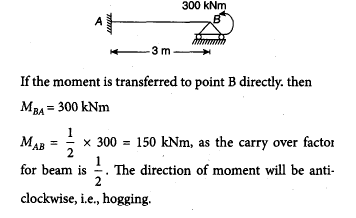QUESTION: 11

The strain energy due to bending in the cantilever beam shown in the figure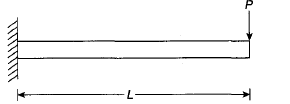Solution: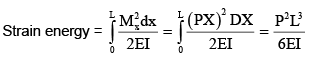QUESTION: 12

In the portal frame shown in the given figure, the ratio of sway moments in column AB and CD will be equal to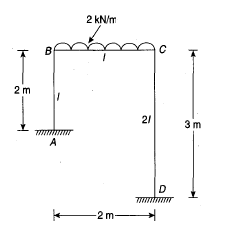Solution: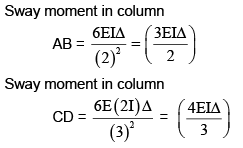Therefore, the ratio of sway moments in columns AB and CD will be equal to 9/8.

QUESTION: 13

The total degree of static indeterminacy of the plane frame shown in the given figure is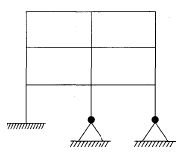Solution: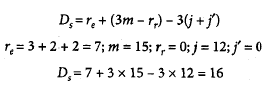QUESTION: 14

The displacement coordinates for a beam are shown in the figure given below. Here, the stiffness matrix will be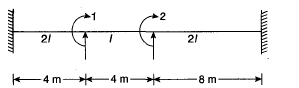Solution: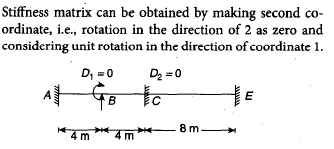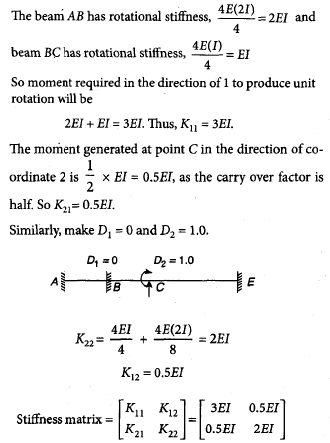QUESTION: 15

The flexibility matrix for the beam shown below is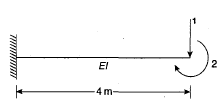Solution: